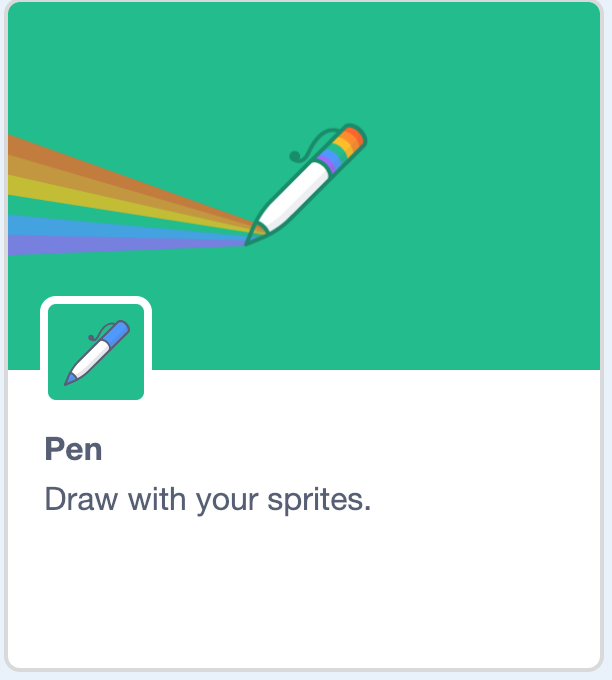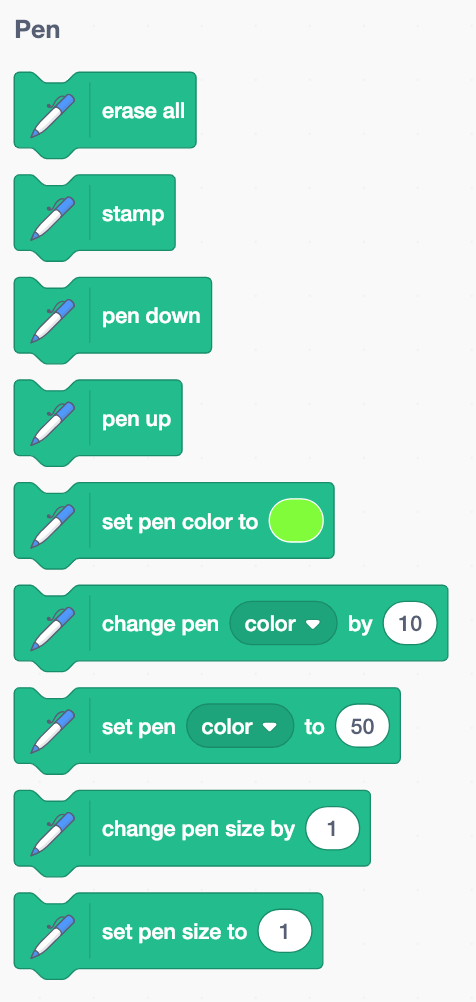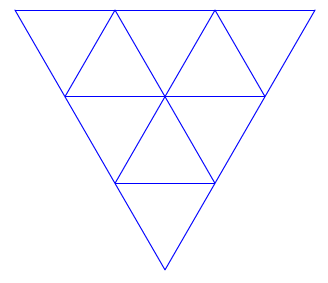Blog of Andrés Aravena
CMB2:

# Homework 1

17 February 2019. Deadline: Friday, 22 February, 9:00.
Updated: 20 February 2019

This week we have two questions. You only need to answer one. If you answer both, it counts as two homework. if you delivered the summer homework you got also one homework.

# Ball hanging from a coil

Modify exercise 3 to include a coil pulling the ball up. The coil pulls the ball upwards, following Hooke’s law. Gravity pulls the ball downwards.

It is not necessary to draw the coil. It is hard to draw it correctly.

You can take a look on the examples we did on classes:

The last one is a good starting point for the answer to this question.

## How to model a coil

You studied coils last semester. You even did an experiment. The result of that experiment was this: the force of a coil depends on

• The elasticity constant
• The initial_length of the coil
• The current length of the coil

According to Hooke’s Law (which you corroborated in your experiment), the force of the coil is force = elasticity*(initial_length - length). Then, using Newton’s second law, we can calculate the acceleration as force/mass.

The suggested values for these variables are:

• elasticity: between 1 and 50. You can use a slider for this.
• initial length: between 10 and 100. You can use a slider for this.
• current length: 180-y position (the coil hangs from the top, at position 180)
• mass: 1000

The speed of the ball will change in each step by the sum of gravity acceleration (-0.1 pointing down) and the coil acceleration (force/mass pointing towards initial_length).

You do not need to draw the coil. You can use pen down to draw the movement of the ball (see below).

# Turtle graphics

Following an ancient tradition, sprites in Scratch can draw lines when they move. To do that, you need to load an extension. First, you have to click in the lower left corner, on a symbol like this:Then you must choose the Pen extension:You can now see new blocks under the Pen section.The essential ones are pen down, pen up and clear. We are going to use them to make some nice figures, like this oneWrite a script that make the cat do the following movements:

• put the cat at coordinates (0,100)
• point in direction 90 degrees
• clean any existing drawing
• put the pen down
• repeat 3 times the following
• repeat 2 times
• turn right 120 degrees
• move 100 steps
• repeat 2 times
• turn left 120 degrees
• move 100 steps
• turn right 120 degrees
• hide the cat

When you finish, share the script and send me the web link (i.e. the URL).